## Class 9 study materials part 7

May 14, 2020NoticeComments Off on Class 9 study materials part 7

Watch the videos and answer the questions

Q.1: In the figure, lines AB and CD intersect at O. If ∠AOC + ∠BOE = 70° and ∠BOD = 40°, find ∠BOE and reflex ∠COE.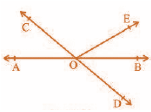Solution:

From the given figure, we can see;

∠AOC + ∠BOE +∠COE and ∠COE +∠BOD + ∠BOE form a straight line.

So, ∠AOC + ∠BOE +∠COE = ∠COE +∠BOD + ∠BOE = 180°

Now, by substituting the values of ∠AOC + ∠BOE = 70° and ∠BOD = 40° we get

∠COE = 110° and

∠BOE = 30°

Q.2: In the Figure, lines XY and MN intersect at O. If ∠POY = 90° and a : b = 2 : 3, find c.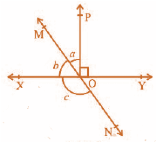Solution:

As we know, the sum of linear pair are always equal to 180°

So,

∠POY + a + b = 180°

substituting the value of ∠POY = 90° (as given in the question) we get,

a + b = 90°

Now, it is given that a : b = 2 : 3 so,

Let a be 2x and b be 3x

∴ 2x + 3x = 90°

Solving this we get

5x = 90°

So, x = 18°

∴ a = 2 × 18° = 36°

Similarly b can be calculated and the value will be

b = 3 × 18° = 54°

From the diagram, b + c also forms a straight angle so,

b + c = 180°

=> c + 54° = 180°

∴ c = 126°

Q.3: In the Figure, POQ is a line. Ray OR is perpendicular to line PQ. OS is another ray lying between rays OP and OR. Prove that ∠ROS = 1/2(∠QOS – ∠POS).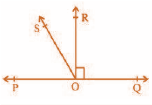Solution:

In the question, it is given that (OR ⊥ PQ) and ∠POQ = 180°

So, ∠POS + ∠ROS + ∠ROQ = 180°

Now, ∠POS + ∠ROS = 180° – 90° (Since ∠POR = ∠ROQ = 90°)

∴ ∠POS + ∠ROS = 90°

Now, ∠QOS = ∠ROQ + ∠ROS

It is given that ∠ROQ = 90°,

∴ ∠QOS = 90° + ∠ROS

Or, ∠QOS – ∠ROS = 90°

As ∠POS + ∠ROS = 90° and ∠QOS – ∠ROS = 90°, we get

∠POS + ∠ROS = ∠QOS – ∠ROS

=>2 ∠ROS + ∠POS = ∠QOS

Or, ∠ROS = ½ (∠QOS – ∠POS) (Hence proved).

Q.4: It is given that ∠XYZ = 64° and XY is produced to point P. Draw a figure from the given information. If ray YQ bisects ∠ZYP, find ∠XYQ and reflex ∠QYP.

Solution: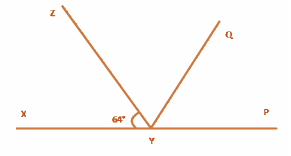Here, XP is a straight line

So, ∠XYZ +∠ZYP = 180°

substituting the value of ∠XYZ = 64° we get,

64° +∠ZYP = 180°

∴ ∠ZYP = 116°

From the diagram, we also know that ∠ZYP = ∠ZYQ + ∠QYP

Now, as YQ bisects ∠ZYP,

∠ZYQ = ∠QYP

Or, ∠ZYP = 2∠ZYQ

∴ ∠ZYQ = ∠QYP = 58°

Again, ∠XYQ = ∠XYZ + ∠ZYQ

By substituting the value of ∠XYZ = 64° and ∠ZYQ = 58° we get.

∠XYQ = 64° + 58°

Or, ∠XYQ = 122°

Now, reflex ∠QYP = 180° + ∠XYQ

We computed that the value of ∠XYQ = 122°. So,

∠QYP = 180° + 122°

∴ ∠QYP = 302°

Q.5: In the Figure, if AB || CD, EF ⊥ CD and ∠GED = 126°, find ∠AGE, ∠GEF and ∠FGE.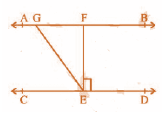Solution:

Since AB || CD GE is a transversal.

It is given that ∠GED = 126°

So, ∠GED = ∠AGE = 126° (As they are alternate interior angles)

Also,

∠GED = ∠GEF + ∠FED

As

EF ⊥ CD, ∠FED = 90°

∴ ∠GED = ∠GEF + 90°

Or, ∠GEF = 126 – 90° = 36°

Again, ∠FGE + ∠GED = 180° (Transversal)

substituting the value of ∠GED = 126° we get,

∠FGE = 54°

So,

∠AGE = 126°

∠GEF = 36° and

∠FGE = 54°

Q.6: In the Figure, if PQ || ST, ∠PQR = 110° and ∠RST = 130°, find ∠QRS.

[Hint : Draw a line parallel to ST through point R.]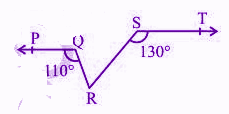Solution: First, construct a line XY parallel to PQ.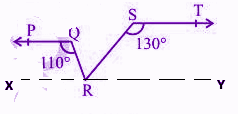As we know, the angles on the same side of transversal is equal to 180°.

So, ∠PQR + ∠QRX = 180°

Or,∠QRX = 180° – 110°

∴ ∠QRX = 70°

Similarly,

∠RST + ∠SRY = 180°

Or, ∠SRY = 180° – 130°

∴ ∠SRY = 50°

Now, for the linear pairs on the line XY-

∠QRX + ∠QRS + ∠SRY = 180°

substituting their respective values we get,

∠QRS = 180° – 70° – 50°

Or, ∠QRS = 60°

Q.7: In Fig. 6.33, PQ and RS are two mirrors placed parallel to each other. An incident ray AB strikes the mirror PQ at B, the reflected ray moves along the path BC and strikes the mirror RS at C and again reflects back along CD. Prove that AB || CD.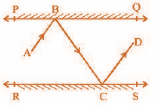Solution:

First, draw two lines BE and CF such that BE ⟂ PQ and CF ⟂ RS.

Now, since PQ || RS,

So, BE || CF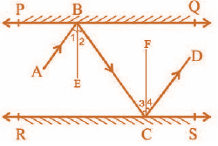As we know,,

Angle of incidence = Angle of reflection (By the law of reflection)

So,

∠1 = ∠2 and

∠3 = ∠4

We also know that alternate interior angles are equal. Here, BE ⊥ CF and the transversal line BC cuts them at B and C

So, ∠2 = ∠3 (As they are alternate interior angles)

Now, ∠1 + ∠2 = ∠3 + ∠4

Or, ∠ABC = ∠DCB

So, AB ∥ CD (alternate interior angles are equal)

Q.8: In Fig. 6.40, ∠X = 62°, ∠XYZ = 54°. If YO and ZO are the bisectors of ∠XYZ and ∠XZY respectively of Δ XYZ, find ∠OZY and ∠YOZ.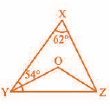Solution:

As we know, the sum of the interior angles of the triangle.

So, ∠X +∠XYZ + ∠XZY = 180°

substituting the values as given in the question we get,

62° + 54° + ∠XZY = 180°

Or, ∠XZY = 64°

Now, As we know, ZO is the bisector so,

∠OZY = ½ ∠XZY

∴ ∠OZY = 32°

Similarly, YO is a bisector and so,

∠OYZ = ½ ∠XYZ

Or, ∠OYZ = 27° (As ∠XYZ = 54°)

Now, as the sum of the interior angles of the triangle,

∠OZY +∠OYZ + ∠O = 180°

substituting their respective values we get,

∠O = 180° – 32° – 27°

Or, ∠O = 121°

Q.9: In the figure, if AB || CD || EF, PQ || RS, ∠RQD = 25° and ∠CQP = 60°, then find ∠QRS?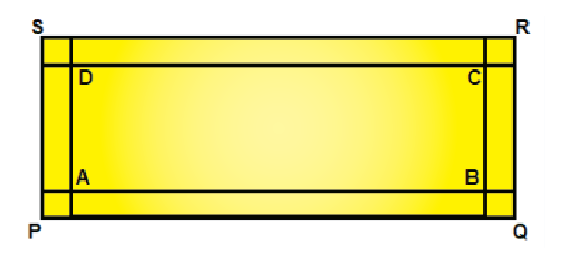Solution:

According to the given figure, we have

AB || CD || EF

PQ || RS

∠RQD = 25°

∠CQP = 60°

PQ || RS.

As we know,

If a transversal intersects two parallel lines, then each pair of alternate exterior angles is equal.

Now, since, PQ || RS

⇒ ∠PQC = ∠BRS

We have ∠PQC = 60°

⇒ ∠BRS = 60° … eq.(i)

We also know that,

If a transversal intersects two parallel lines, then each pair of alternate interior angles is equal.

Now again, since, AB || CD

⇒ ∠DQR = ∠QRA

We have ∠DQR = 25°

⇒ ∠QRA = 25° … eq.(ii)

Using linear pair axiom,

We get,

∠ARS + ∠BRS = 180°

⇒ ∠ARS = 180° – ∠BRS

⇒ ∠ARS = 180° – 60° (From (i), ∠BRS = 60°)

⇒ ∠ARS = 120° … eq.(iii)

Now, ∠QRS = ∠QRA + ∠ARS

From equations (ii) and (iii), we have,

∠QRA = 25° and ∠ARS = 120°

Hence, the above equation can be written as:

∠QRS = 25° + 120°

⇒ ∠QRS = 145°

### Class 9 Maths Chapter 6 Extra Questions

1. If two lines intersect, prove that the vertically opposite angles are equal.
2. Bisectors of interior ∠B and exterior ∠ACD of a Δ ABC intersect at the point T.Prove that ∠ BTC = ½ ∠ BAC.
3. A transversal intersects two parallel lines. Prove that the bisectors of any pair of corresponding angles so formed are parallel.
4. In the figure, OD is the bisector of ∠AOC, OE is the bisector of ∠BOC and OD ⊥ OE. Show that the points A, O and B are collinear.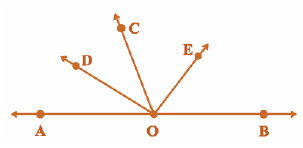5. The angles of a triangle are in the ratio 5 : 3: 7. The triangle is

• An acute-angled triangle
• An obtuse-angled triangle
• A right triangle
• An isosceles triangle

6. Can a triangle have all angles less than 60°? Give reason for your answer.

7. Can a triangle have two obtuse angles? Give reason for your answer.

8. How many triangles can be drawn having its angles as 45°, 64° and 72°? Give reason for your answer.

9. How many triangles can be drawn having its angles as 53°, 64° and 63°? Give reason for your answer.

Question 1:
(a) Name the plant tissue which is found in the husk of a coconut. Identify the chemical which is responsible for its stiffness.
(b) Give one way in which it differs from parenchymatous cells.
(a) Sclerenchyma fibres, lignin is responsible for its stiffness.
(b) Scelerenchyma are thick walled dead cells while parenchyma are thin walled living cells.

Question 2:
(a) Name the living component which is common in both the complex permanent tissue found in the plants. What is its function?
(b) Give any two ways in which this tissue differs functionally from each other.
(a) Parenchyma. It takes part in the storage of nutrients and slows down lateral conduction (water in xylem and nutrients in phloem).
(b) Xylem:
(i) Xylem conducts water and minerals.
(ii) Conduction is unidirectional, upwardly from roots to stem tips and leaves.
Phloem:
(i) Phloem conducts food materials.
(ii) It is bidirectional from leaves to roots and from roots to stem tips.

Define the term ‘tissue’?
how many types of elements together make up the xylem tissue? Name them.
What is the specific function of cardiac muscle?

Problem 1.
Choose the right Solution from the four alternatives given below

(i) Which one of the following describes the drainage patterns resembling the branches of a tree?

(b) Dendritic
(c) Centrifugal
(d) Trellis

Solution (b)

(ii) In which of the following states is the Wular Lake located ?
(a) Rajasthan
(b) Punjab
(d) Jammu and Kashmir

Solution (d)

(iii) The river Narmada has its source as
(a) Satpura
(b) Amarkantak
(c) Brahmagiri
(d) Slopes of the Western Ghats

Solution (b)

(iv) Which one of the following lakes is a salt water lake?
(a) Sambhar
(b) Wular
(c) Dal
(d) Gobind Sagar

Solution (a)

(v) Which one of the following is the longest river of peninsular India?
(b) Krishna
(c) Godavari

Solution (c)

(vii) Which one amongst the following rivers flow through a Rift Valley?
(b) Krishna
(d) Tapi

Solution (d)

Problem 2. Solution the following Problem s briefly.

(i) What is meant by a water divide? Give an example

Solution Any elevated area such as a mountain or an upland that separate two drainage basins is called a water divide. An example are the Western Ghats.

(ii) Which is the largest river basin in India?

Solution The Ganga Basin is the largest river basin in India.

(iii) Where do the rivers Indus and Ganga have their origin?

Solution The river Indus rises in Tibet. near lake Mansarovar. and the Ganga originates at the Gangotri Glacier. Both of them have their origin in the Himalayas.

(iv) Name the two headstreams of the Ganga. Where do they meet to form the Ganga?

Solution The two head streams of the Ganga are the Bhagirathi and Alaknanda. They meet at Devprayag in Uttarakhand to form the Ganga.

(v) Why does the Brahmaputra in its Tibetan part have less silt, despite a longer course?

Solution In TIbet. the river Indus known as Tsang Po carries a smaller volume of water and less silt as it is a cold and dry area.

In India. the river carries a large volume of water and considerable amount of silt because it passes through a region of high rainfall.

(vi) Which two peninsular rivers flow through a trough ?

Solution Narmada and Tapi are two peninsular rivers, which flow through a trough.

(vii) State some economic benefits of rivers and lakes.

Solution Lakes

• Lakes are of a great value to human beings.
• Lakes help to regulate the flow of rivers.
• Lakes help to prevent flooding during rainy season.
• During the dry season, lakes help to maintain an even flow of water.
• Lakes can also be used for developing hydel power.
• They moderate the climate of the surroundings and maintain the aquatic ecosystem.
• They enhance natural beauty and help to develop tourism and provide recreation. e.g., Dal Lake and Naini Lake at Nainital.
• Lakes provide opportunities for fishery development.

Rivers

• They help to develop hydel power.
• They provide water for irrigation, for drinking and other requirements.
• They help to develop fisheries.

Problem 3. Below are given names of a few lakes of India. Group them under two categories natural and created by human beings.

Problem 4. Discuss the significant difference between the Himalayan and the peninsular rivers.

Problem 5. Compare the east flowing and west flowing rivers of the Peninsular plateau.

problem 6. Why are rivers important for the country’s economy?

Solution Rives are important for the country’s economy because water from the rivers is a basic natural resource essential for various human activities. These are

1. The rivers provide water for irrigation.
2. They provide fertility to the soil.
3. They are useful for navigation
4. They help to generate hydro-electricity.
5. They help to develop tourism.
6. They provide water for various domestic uses.
7. They provide livelihood to fishermen.
8. They help to moderate the climate and environment of nearby areas